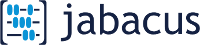version 0.3
April 1, 2020

## Timber Bolted Connectionversion 0.3
April 1, 2020

#### Members

Number of members:

#### Side Member(s)

Material:
Thickness:
mm
Angle relative to grain:
deg
mm
mm

#### Main Member

Material:
Thickness:
mm
Angle relative to grain:
deg
mm
mm

#### Bolts

Bolt diameter:
Yield strength, fy:
MPa
Ultimate strength, Fu:
MPa
Bolt rows are parallel to the applied load
Number of Rows:
Bolts Per Row:
Row Spacing:
mm
Bolt Spacing in row:
mm

#### Conditions

Wood:
Service:
Treatment:
waiting for input...

#### Parallel to Grain - Lateral Resistance

(Both members loaded parallel to grain)
&Phi = 0.7
KT = 1.0
JG = 1
Joint detail = A
KD = 1
JR = 1
Bolt d = 9.525 mm
KSF = 1
JL = 1
JF = JG*JL*JR = 1
pu calculations
Gside = 0.49
f2 main = 27.93
(c) = n/a
(f) = 7577 N
ns = 1
f1 side = 27.93
(b) = 29795 N
(e) = 7909 N
pu = 3567 N
Gmain = 0.49
(a) = 8087 N
(d) = 3567 N
(g) = 3899 N
nf = 1
Pu=pu*KD*KSF*KT = 3.57 kN

#### Perpendicular to Grain - Lateral Resistance

(Side member parallel to grain and main member perpendicular to grain)
&Phi = 0.7
KT = 1.0
Joint detail = A
KD = 1
Bolt d = 9.525 mm
KSF = 1
qu modes
Gside = 0.49
f2 main = 12.15
(c) = n/a
(f) = 4209 N
ns = 1
f1 side = 27.93
(b) = 12959 N
(e) = 7477 N
qu = 3036 N
JR = 1
Gmain = 0.49
(a) = 8087 N
(d) = 3135 N
(g) = 3036 N
nf = 1
Qu=qu*KD*KSF*KT = 3.04 kN

#### Loads at Angle - Lateral Resistance

Main member parallel to grain, Side member perpendicular to grain
Qr = 1.55 kN
Nr side = 2.5 kN
Main member perpendicular to grain, Side member parallel to grain
Qr = 2.13 kN
Nr_ main = 2.13 kN

#### Nr = Pr*Qr/(Pr*sin2&Theta + Qr*cos2&Theta) =2.13 kN

By using Jabacus or information derived from this Service, you have agreed to the Terms of Service.

This information must be read or used in conjunction with the 2005 reprint of CAN/CSA O86-01 Engineering Design in Wood.
In the event of discrepancies, CAN/CSA O-86 shall govern.